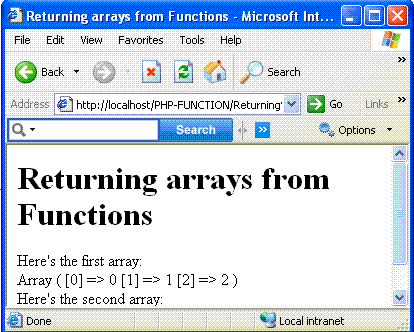# PHP Programing language

Which Can Returning Arrays for Function
 Previous Home Next
You can Return arrays from function as easily as you return simple values. Say you have a function ,create_array, that creates arrays of the length you specify by passing a number to the create_array function. This function stores 0 in the zeroth element,1 in the first element, and so on.

Here's how you can create the array,\$ array, to return form this function:
that creates the array named \$array. Now you can return it from the create_array function just as ou would return any simple value-with the return statement:

```function create_array (\$number)
{
for (\$loop_counter = 0; \$loop_counter <
\$number; \$loop_counter++)
{
\$array[] = \$loop_counter;
}
return \$array;
}
```
Here's how you can put the create_array function to work in an example.
```<html>
<title>Returning arrays from Functions  </title>
<body>
<h1>Returning arrays from Functions </h1>
<?php
\$data = create_array(3);
echo "Here's the first array:<br>";
print_r(\$data);
echo "<br>";
\$data_2 = create_array(4);
echo "Here's the second array:<br>";
print_r(\$data_2);

function create_array (\$number)
{
for (\$loop_counter = 0; \$loop_counter <
\$number; \$loop_counter++)
{
\$array[] = \$loop_counter;
}
return \$array;
}
?>
</body>
</html>
```

Output:Previous Home Next